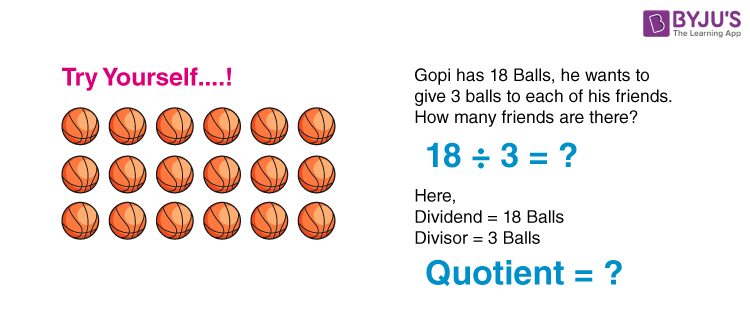# Quotient

In Maths, the quotient is the number which is generated when we perform division operations on two numbers. Basically, it is the result of the division method. There are four main terminologies used in the arithmetic division such as divisor, dividend, quotient and remainder. Each term will be explained here in this article with meaning and examples. Also, learn how to divide numbers here

## How to get Quotient?## Quotient Meaning

In the division method, a number is divided by another number to get a different number as an output. Here, the number/integer which is getting divided is known as a dividend and the integer which divides a given number is the divisor. The divisor which does not divide a number entirely gives a number, which is said to be the remainder. The division symbol is denoted by ‘÷’ or ‘/’. So, we can represent the division method as;

 Dividend = Quotient × Divisor + Remainder

If the remainder is equal to 0, then;

 Dividend = Quotient × Divisor

Therefore,

 Quotient = Dividend ÷ Divisor

## Parts of Division Problem

We can represent the division operation in the form of;

 Dividend ÷ Divisor = Quotient and Remainder

When a dividend is divided by a divisor, we get the result. So, basically, the division is the inverse process of multiplication, such that when we multiply the quotient and divisor, we will get the dividend. Thus we can also define divisor, dividend and remainder here.

• Dividend: The number which is required to be divided
• Divisor: The number which divides the given dividend
• Remainder: The number which is left after the division method. When a number is completely divided then the remainder is zero, but when a number is partially divided, the remainder is not equal to zero.

## Quotient Representation

The quotient has extensive use throughout Maths and is usually referred to as a fraction or a ratio. Let us see it’s representation to understand it better.In the above representation, we can see the numerator is the dividend and the denominator is the divisor. When the numerator is divided by the denominator, the result is the quotient. Now let us see the representation in terms of the long division method in the below figure.## How to Determine the Quotient of a Number?

While working with division problems, first we have to determine which is the dividend and divisor. To get the quotient of a number, the dividend is divided by the divisor. It means that the problem should be in the form:

Dividend (obelus sign) Divisor (equal to sign) = Quotient

(i.e.) Dividend ÷ Divisor = Quotient

If a dividend is perfectly divided by divisor, we don’t get the remainder (Remainder should be zero). If the dividend is not perfectly divided by the divisor, we get a remainder.

For example, 12 ÷ 2 = 6

In this case, the dividend 12 is perfectly divided by 2. So we get the quotient value as 6 and remainder 0.

Now, let us consider the other example, 15 ÷ 2. In this case, 15 is not exactly divisible by 2, hence we get the quotient value as 7 and remainder 1.

### Quotient Examples

Q.1: Divide 24 by 4.

Solution: 24 ÷ 4 = 6

Q.2: Find the quotient for 105÷5.

Solution: 105÷5 = 21

Hence, 21 is the quotient

Q.3: Divide 60.5÷5.

Solution: 60.5÷5 = 60.5/5 = 12.1

Q.4: Solve 108/12.

Solution: 108/12 = 9

Hence, the quotient is 9.

Q.5: Write the quotient and remainder for the following:

Solution:

 Division Quotient Remainder 114÷12 9 6 32/6 5 2 125/9 13 8

### Practice Questions

• Divide 177 by 3.
• Divide 800/4.
• Find the value of 45/12.
• Find 63÷9.

## Frequently Asked Questions on Quotient

Q1

### What is meant by quotient?

In Maths, a quotient is a resultant number when one number is divided by the other number. In other words, a dividend is divided by a divisor, we get the result quotient.

Q2

### What are the different parts of the division problem?

The different parts of the division problem are:
Dividend
Divisor
Quotient
Remainder

Q3

### Is quotient should be the answer to the division problem?

Yes, the quotient should be the solution to any division problem. If a number 5 is divided by 5, we get the quotient 1, which is the answer to the division problem.

Q4

### What is the quotient of 17 divided by 3?

If 17 is divided by 3, we get the quotient 5 and remainder 2. (i.e) 17(Dividend) ÷ 3 (Divisor) = 5 (Quotient) + 2 (Remainder).

Q5

### What is the quotient formula?

The quotient formula is given as follows:
Dividend ÷ Divisor = Quotient (if the remainder is zero)
The general formula for any division problem is given by:
Dividend ÷ Divisor = Quotient + Remainder.